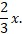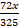• Save

# Partnership Problems with Solutions for SSC and Bank Exams

Vikram Singh11 months ago 8.6K ViewsMost of the students have the question that how to solve partnership problems for competitive exams. If you have also the same question in your mind, you can learn here partnership problems with solutions for ssc and bank exams in this post. These partnership questions are very important which asked often in competitive exams. Get the latest partnership questions with answers in both Hindi and English languages.

You should practice with the help of these examples and solution for your preparation. To more practice with questions and answers visit these selective partnership questions and answers and increase your performance level with different equations.

## Partnership Problems with Solutions for Competitive Exams

Q.1. A, B and C started a business by investing Rs. 1,20,000, Rs. 1,35,000 and Rs. 1,50,000 respectively. Find the share of each, out of an annual profit of Rs. 56,700.

Solution:

Ratio of shares of A,B and C = Ratio of their investments

= 120000 : 135000  :  150000 = 8 : 9 : 10.

$$∴ A's \ share = Rs. \left(56700×{8\over27}\right)= Rs. 16800.$$

$$B's \ share = Rs. \left(56700×{9\over27}\right)= Rs. 18900.$$

$$C's \ share = Rs. \left(56700×{10\over27}\right)= Rs.21000.$$

Q.2. Alfred started a business investing Rs. 45,000. After 3 months, Peter joined him with a capital of Rs. 60,000. After another 6 months, Ronald joined them with a capital of Rs. 90,000. At the end of the year, they made a profit of Rs. 16,500. Find the share of each.

Solution:

Clearly, Alfred invested his capita for 12 months, Peter for 9 months and Ronald for 3 months.

So ratio of their capitals = (45000×12) : (60000×9) : (90000×3)

= 540000 : 540000 : 270000 = 2 : 2 : 1

$$∴ Alfred's\ share = Rs. \left(16500×{2\over5}\right)= Rs.6600;$$

$$Peter's \ share = Rs. \left(16500×{2\over5}\right)= Rs. 6600;$$

$$Ronald's \ share = Rs. \left(16500×{1\over5}\right)= Rs.3300;$$

Q.3. A, B and C start a business each investing Rs. 20,000. After 5 months A withdrew Rs.5000, B withdrew Rs. 4000 and C invests Rs. 6000 more. At the end of the year, a total profit of Rs. 69,900 was recorded. Find the share of each.

Solution:

Ratio of the capitals of A, B and C

= 20000×5+15000×7 : 20000×5+16000×7: 20000×5+26000×7

= 205000 : 212000 : 282000 = 205 : 212 : 282.

$$∴ A's\ share = Rs. \left(69900×{205\over699}\right)= Rs.20500;$$

$$B's \ share = Rs. \left(69900×{212\over699}\right)= Rs. 21200;$$

$$C's \ share = Rs. \left(69900×{282\over699}\right)= Rs.28200;$$

Q.4. A, B and C enter into partnership. A invests 3 times as much as B invests and invests two-thirds of what C invests. At the end of the year, the profit earned is Rs. 6600. What is the share of B?

Solution:

Let C’s capital = Rs. x. Then, B, capital = Rs.$$A's\ capital = Rs. \left(3×{2\over3}x\right)= Rs.2x.$$

$$= 2x : {2\over3}x:x=6:2:3.$$

$$Hence, B's \ share = Rs.\left(6000×{2\over11}\right)=Rs.1200.$$

Q.5. Four milkman rented a pasture. A grazed 24 cows for 3 months, B 10 cows for 5 months; C 35 cows for 4 months and D 21 cows for 3 months.If A’s share of rent is Rs. 720, find the total rent of the field.

Solution:

Ratio of shares of A, B, C, D = (24×3) : (10×5) : (35×4) : (21×3)

= 72 : 50 : 140 : 63.

Let total rent be Rs. x. Then, A’s share = Rs..

$$∴ {72x\over325}=720↔x={720×325\over72}=3250$$

Hence, total rent of the field is Rs. 3250.

For more Partnership questions and answers, Visit next page..